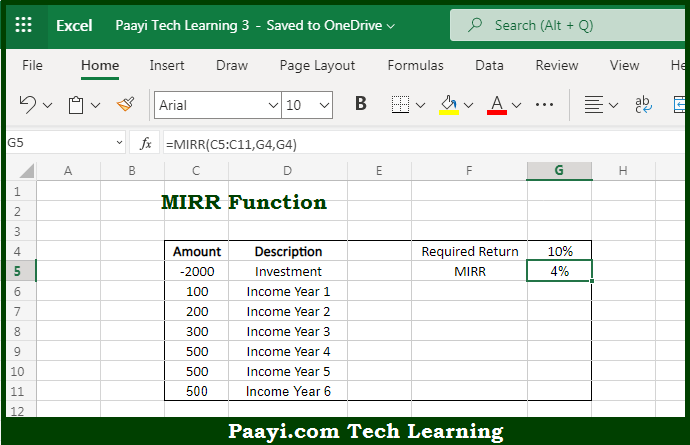# Learn How to Use Microsoft Excel MIRR Function

Written by | 0 Comments | 489 Views

In this article, you will learn how to use the Microsoft Excel MIRR function and its prime function in Microsoft Excel. You will also get to know the Microsoft Excel MIRR function return value and syntax with the help of some examples.

Microsoft Excel MIRR Function

The main purpose of the Microsoft Excel MIRR function is to calculate the modified internal rate of the return. That implies, with the help of the MIRR function you can able to return the modified internal rate of return, which is abbreviated as MIRR, for a series of cash flows, it should be noted that both discount rate and reinvestment rate for future cash flows. So, with the help of the MIRR function, you can able to calculate the modified internal rate of return.

Return Value of MIRR Function

The return value will be calculated returns as a percentage.

Syntax of MIRR Function

=MIRR(values, finance-rate, investment-rate)

Where the arguments:

• values: This is the array or reference to cells containing cash flows.
• finance-rate: This is the required rate of return or discount rate in the form of a percentage.
• investment-rate: This is the interest rate received on cash flows reinvested as a percentage.

How to Use Microsoft Excel MIRR Function?So we know that Microsoft Excel MIRR function you can able to calculate a modified internal rate of the return. That implies, with the help of the MIRR function you can able to return the modified internal rate of return, which is abbreviated as MIRR, for a series of cash flows, it should be noted that both discount rate and reinvestment rate for future cash flows. So, with the help of the MIRR function, you can able to calculate the modified internal rate of return.